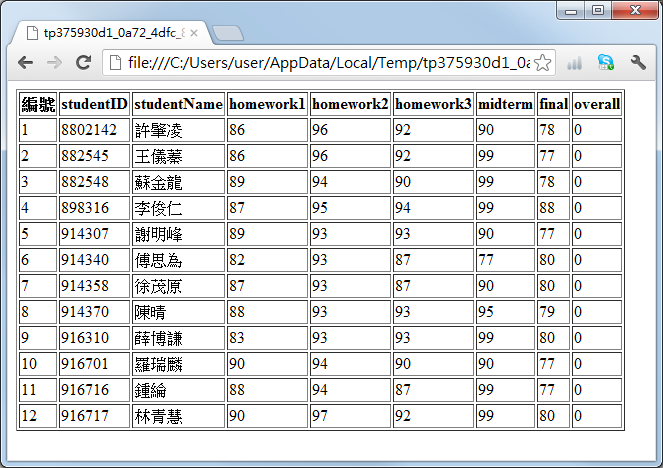## 13-4 雿輻鍂MATLAB撠滩??坔澈?脰??亥岷

1. 設定 DSN，讓 MATLAB 能經由 DSN 及 ODBC 來操控資料庫。（此部分請見上一節的說明）
3. 使用 database 指令來連結至資料庫，其格式如下：
6. 使用 ping 指令來檢查資料庫連結狀態，例如 ping(conn)。這步驟純粹是為了檢查資料庫連結狀態，在一般程式碼中，可以省略。
7. 使用 exec 指令來執行 SQL 命令，並傳回 cursor 物件，以邊開始抓取資料。其格式如下：
8. cursor = exec(conn, sqlCommand)
9. 其中 conn 是在步驟 3 所設定的 資料庫連結物件，sqlCommand 則是欲執行的 SQL 命令，所傳回的 cursor 物件，代表此次執行 SQL命令的管道或溝通方式，我們可以根據此管道來取得所有的資料。
10. 可以使用 setdbprefs 指令來設定回傳資料的格式，一般是異質陣列（預設值）或是結構陣列，詳見後面的範例。
11. 使用 fetch 指令來抓取資料，例如 cursor = fetch(cursor, 10) 會抓取10筆資料，並將資料存放在 cursor 物件中的 Data 欄位。
12. 將 cursor 物件的資料傳至 MATLAB 變數，例如：songData = cursor.Data。
13. 可以反覆使用 cursor 物件來取得下達 SQL 命令所得到的所有資料。
14. 最後可以使用 close 指令來關閉 cursor 物件及資料庫連結。

Example 1: 13-與資料庫的整合/getData01.mdsn = 'dsnSong01'; % 設定資料來源名稱（指到 song01.mdb） logintimeout(5); % 設定嘗試連結資料庫的時間 conn = database(dsn, '', ''); % 連結資料庫 sql = 'select * from song'; % 執行設定 SQL 命令 cursor = exec(conn, sql); % 執行 SQL 命令，並傳回 cursor 物件 cursor = fetch(cursor, 8); % 經由 cursor 物件，抓取 8 筆資料 songData = cursor.data % 將資料傳至 MATLAB 變數 songData close(cursor); % 結束 cursor 物件 close(conn); % 結束資料庫連結songData = [ 1] '用心良苦' '張宇'  [ 2] '聽海' '張惠妹'  [ 4] '牽手' '張惠妹'  [ 6] '最熟悉的陌生人' '蕭亞軒'  [ 8] '戀人未滿' 'S.H.E'  [ 9] 'I.O.I.O.' 'S.H.E'   '每次都想呼喊你的名字' '永邦'   '最熟悉的陌生人' '蕭亞軒' 

Example 2: 13-與資料庫的整合/getDataAttr01.mdsn = 'dsnSong01'; % 設定資料來源名稱（指到 song01.mdb） logintimeout(5); % 設定嘗試連結資料庫的時間 conn = database(dsn, '', ''); % 連結資料庫 sql = 'select * from song'; % 設定 SQL 命令 cursor = exec(conn, sql); % 執行 SQL 命令，並傳回 cursor 物件 cursor = fetch(cursor, 10); % 經由 cursor 物件，抓取 10 筆資料 fprintf('資料筆數 = %d\n', rows(cursor)); % 顯示資料筆數 fprintf('欄位個數 = %d\n', cols(cursor)); % 顯示欄位個數 fprintf('欄位名稱 = %s\n', columnnames(cursor));% 顯示資料庫欄位名稱 fprintf('欄位寬度 = %d\n', width(cursor, 3)); % 顯示第三個欄位的寬度 attributes = attr(cursor, 3) % 顯示第三個欄位的所有資訊 close(cursor); % 結束 cursor 物件 close(conn); % 結束資料庫連結資料筆數 = 10 欄位個數 = 4 欄位名稱 = '序號','歌曲名稱','主唱者','年份' 欄位寬度 = 255 attributes = fieldName: '主唱者' typeName: 'VARCHAR' typeValue: 12 columnWidth: 255 precision: [] scale: [] currency: 'false' readOnly: 'false' nullable: 'true' Message: []

Example 3: 13-與資料庫的整合/getData02.mdsn = 'dsnScore01'; % 設定資料來源名稱（指到 score01.mdb） logintimeout(5); % 設定嘗試連結資料庫的時間 conn = database(dsn, '', ''); % 連結資料庫 sql = 'select * from score'; % 執行設定 SQL 命令 cursor = exec(conn, sql); % 執行 SQL 命令，並傳回 cursor 物件 setdbprefs('DataReturnFormat', 'structure'); % 設定 cursor 傳回資料格式是結構陣列 cursor = fetch(cursor, 10); % 經由 cursor 物件，抓取 10 筆資料 score = cursor.Data % 經資料設定至 score 變數 close(cursor); % 結束 cursor 物件 close(conn); % 結束資料庫連結 setdbprefs('DataReturnFormat', 'cellarray'); % 改回預設的資料格式score = studentID: {10x1 cell} studentName: {10x1 cell} homework1: [10x1 double] homework2: [10x1 double] homework3: [10x1 double] midterm: [10x1 double] final: [10x1 double] overall: [10x1 double]

Hint

Example 4: 13-與資料庫的整合/db2struct01.mDSN = 'dsnScore01'; % 設定資料來源名稱（指到 score01.mdb） logintimeout(5); % 設定嘗試連結資料庫的時間 conn = database(DSN, '', ''); % 連結資料庫 sql = 'select * from score order by studentID'; % 設定 SQL 命令 cursor = exec(conn, sql); % 執行 SQL 命令，並傳回 cursor 物件 cursor = fetch(cursor); % 經由 cursor 物件，抓取全部資料 score = cursor.data % 將資料傳至 MATLAB 變數 scoregData temp = columnnames(cursor); % 顯示資料庫欄位名稱 eval(['fieldNames = {', temp, '}'';']); % 將欄位名稱指定到 fieldNames 變數 score2 = cell2struct(score, fieldNames, 2) % 將異質陣列 score 轉成結構陣列 score2 close(cursor); % 結束 cursor 物件 close(conn); % 結束資料庫連結score = '8802142' '許肇凌'       '882545' '王儀蓁'       '882548' '蘇金龍'       '898316' '李俊仁'       '914307' '謝明峰'       '914340' '傅思為'       '914358' '徐茂原'       '914370' '陳晴'       '916310' '薛博謙'       '916701' '羅瑞麟'       '916716' '鍾綸'       '916717' '林青慧'       score2 = 12x1 struct array with fields: studentID studentName homework1 homework2 homework3 midterm final overall

1. 使用MATLAB的「陣列編輯器」（Array Editor）來檢視。例如執行過上述範例後，可以直接在MATLAB輸入 open score 或 open score2，就可以直接檢視陣列內容，也可以修改。
2. 也可以將資料寫到網頁，以便上下捲動觀看。

Example 5: 13-與資料庫的整合/db2struct02.mDSN = 'dsnScore01'; % 設定資料來源名稱（指到 score01.mdb） logintimeout(5); % 設定嘗試連結資料庫的時間 conn = database(DSN, '', ''); % 連結資料庫 sql = 'select * from score order by studentID'; % 設定 SQL 命令 cursor = exec(conn, sql); % 執行 SQL 命令，並傳回 cursor 物件 cursor = fetch(cursor); % 經由 cursor 物件，抓取全部資料 score = cursor.data; % 將資料傳至 MATLAB 變數 score temp = columnnames(cursor); % 顯示資料庫欄位名稱 eval(['fieldNames = {', temp, '}'';']); % 將欄位名稱指定到 fieldNames 變數 score2 = cell2struct(score, fieldNames, 2); % 將異質陣列 score 轉成結構陣列 score2 close(cursor); % 結束 cursor 物件 close(conn); % 結束資料庫連結 struct2html(score2); % 顯示結構陣列 score2 於瀏覽器MATLAB程式設計：進階篇